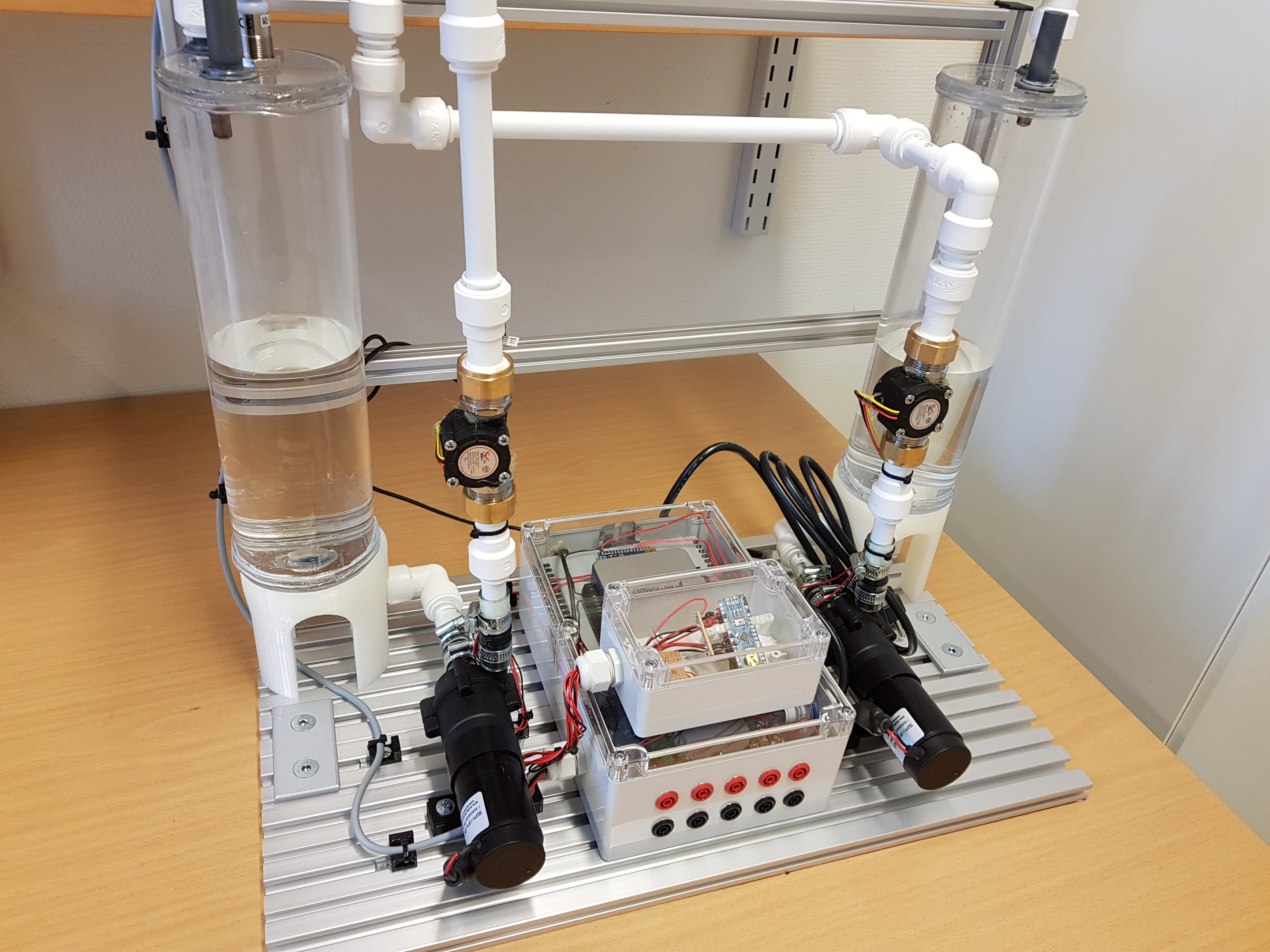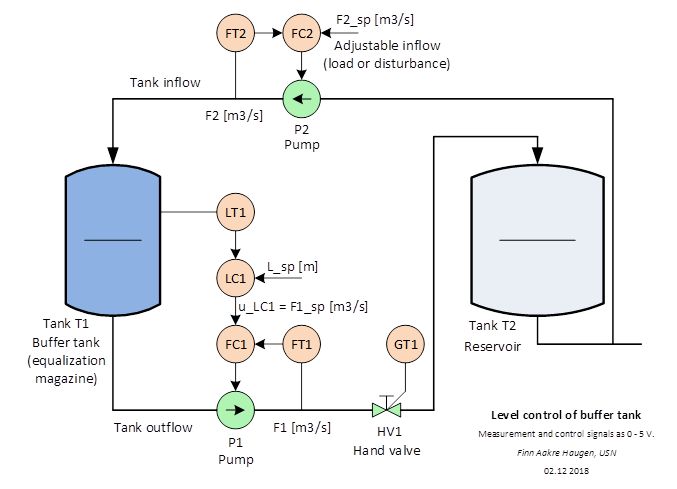# Lab Station: Buffer tank

University of South-Eastern Norway (campus Porsgrunn) has 18 laboratory buffer tanks, see Figure 1. The tanks are used in control courses in both bachelor and master programmes in technology.Figure 1: Buffer tank

## Technical description

Figure 2 shows a piping and instrumentation diagram (P&I D) of the tank including a level control system.Figure 2: Piping and instrumentation diagram (P&I D) of the buffer tank a level control system.

The measurement signals (analog inputs) from the sensors LT1, FT1 and FT2 are voltage signals in the range 0 – 5 V.

The control signals (analog outputs) to the actuators pump P1and pump P2 are voltage signals in the range 0 – 5 V.

A built-in NI USB-6001 IO device handles the IO. This IO device can be connected to a PC with a USB cable.

As an alternative to using the NI USB-6001 IO device, there are terminals on the rig for direct analog IO.

To appear.

To appear.

## Level controller

The control signal generated by the level controller is a demanded outflow:

u = F_out

The level controller may be a PI controller or some other controller.

## Mathematical model

Mass balance of the water in the tank:

rho*A*dh_dt = rho*F_in – rho*F_out

where:

·       h [cm] is the water level in the tank.

·       F_in [cm^3/s] is water inflow.

·       F_out [cm^3/s] is water outflow.

·       A [cm^2] is cross-sectional area of tank.

·       rho [g/cm^3] is water density.

Cancelling out rho and solving for dh_dt gives the

dh_dt = (1/A)*(F_in - F_out)

Nominal values and ranges:

·       h_nom = 15. Range: 0 - 30.

·       F_in_nom = 50. Range: 0 - 100.

·       F_out_nom = 50. Range: 0 - 100.

·       A = 56.7.

·       rho = 1 (but its value is not needed).

## How realize white noise in a simulator

If you want to include white process disturbance (noise) or white measurement noise in a simulator of the buffer tank programmed in Python, you may use the following information about how to generate uniformly distributed white noise with specified standard deviation:

std_noise = 0.01  # Standard deviation. 0.01 is an example value. Select any other.
var_noise = std_noise**2  # Variance
ampl_noise = np.sqrt(3*var_meas_noise)  # Ampl of uniformly dist white noise. (np is numpy.)
v_k = np.random.uniform(-ampl_noise, ampl_noise, 1)  # The noise (point value)